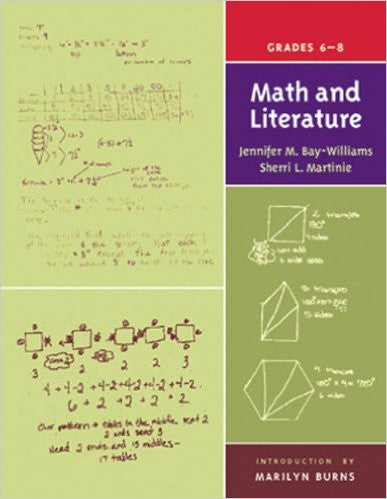# Math And Literature Grade 6 - 8

Rs. 599.00 Rs. 799.00

From Quack and Count to Harry Potter, the imaginative ideas in children's books come to life in math lessons through this unique series. Each resource provides more than 20 classroom-tested lessons that engage children in mathematical problem solving and reasoning. Each lesson features an overview, materials required, and a vignette of how the lesson actually unfolded in a classroom. This book includes a reference chart indicating the mathematical concept each lesson covers, such as number, geometry, patterns, algebra, measurement, data analysis, or probability.

Publisher: Math Solutions
Author: Jennifer M. Bay-Williams
ISBN: 9780941355636
Pages: 169
Format: Paperback
Dimensions: 8.5 x 0.6 x 11 inches